edHelper subscribers - Create a new printable

Number of Keys
 Select the number of different printables: 1 key 2 keys 3 keys 4 keys 5 keys

Number of Pages (for each key)
 Select the number of pages: 1 page 2 pages 3 pages 4 pages

Include an answer key (answer keys will be at the end of the printable)
High School Geometry
High School Geometry
Math

 Name _____________________________Date ___________________ (Key 1 - Answer ID # 0656487)
1.

Find the perimeter of a rhombus with diagonals 6 and 18.

2.

In rhombus ABCD, AB=(8x - 11) and BC=(3x + 49). Find the perimeter.

3.

An equilateral quadrilateral must be a _____.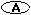rectangle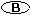isosceles trapezoid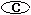square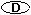rhombus

4.

In rhombus ABCD, the bisectors of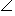B andC must be ______.parallelcongruentsupplementsperpendicular

5.

Given a quadrilateral ABCD, find the midpoint of each side. The midpoints of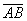,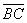,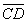, and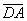are E, F, G, and H respectively. What type of quadrilateral must EFGH be?a rhombusa rectanglea parallelograman isosceles trapezoid

6.

Which of the following statements is NOT true?A square is a rhombus with congruent diagonals.A square is a regular quadrilateral.A rhombus is an equiangular parallelogram.A quadrilateral is a rectangle if and only if all angles are congruent.

7.

In quadrilateral ABCD,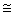and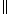. ABCD must be a ______.trapezoidrectangleparallelogramrhombus

8.

The diagonals of a ______ are perpendicular bisectors of each other.rectangletrapezoidrhombusparallelogram

9.

Which of the following is NOT true?A rectangle is a parallelogram.A rectangle is a rhombus.A square is a rhombus.A square is a rectangle.

10.

Which of the following is NOT a property of a rectangle?Diagonals bisect its angles.All angles are right angles.Opposite sides are congruent.Diagonals bisect each other.

edHelper subscribers - Create a new printable

Number of Keys
 Select the number of different printables: 1 key 2 keys 3 keys 4 keys 5 keys

Number of Pages (for each key)
 Select the number of pages: 1 page 2 pages 3 pages 4 pages